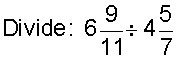# Challenge Exercises: Multiplying and Dividing Fractions and Mixed Numbers

Directions: Perform the necessary operation in each exercise below. Be sure to simplify your result, if necessary. Click once in an ANSWER BOX and type in your answer; then click ENTER. After you click ENTER, a message will appear in the RESULTS BOX to indicate whether your answer is correct or incorrect. To start over, click CLEAR.

Note: To write the fraction three-fourths, enter 3/4 into the form. To write the mixed number four and two-thirds, enter 4, a space, and then 2/3 into the form.

 1. A candy bar is 9/15 of an inch long. If it is divided into pieces that are 1/5 of an inch long, then how many pieces is that? ANSWER BOX:   pieces   RESULTS BOX:
 2. Justin gave 2/3 of his pizza to 8 friends who shared the pizza equally. What fraction of the original pizza did each friend get? ANSWER BOX:   RESULTS BOX:
 3.ANSWER BOX:   RESULTS BOX:
 4. Marcy's garden is 3 and 4/5 meters long and 2 and 7/8 meters wide. What is the area of the garden? ANSWER BOX:  m2   RESULTS BOX:
 5.ANSWER BOX:   RESULTS BOX:
 6. A chocolate bar is 11/12 of an inch long. If it is divided into pieces that are 5/8 of an inch long, then how many pieces is that? ANSWER BOX:   pieces   RESULTS BOX:
 7. A painter had a trough with 22 liters of paint. If each bucket holds 2 and 3/4 liters, then how many buckets of paint can be poured from the trough? ANSWER BOX:   buckets   RESULTS BOX:
 8.ANSWER BOX:   RESULTS BOX:
 9. Frank has 8 and 2/3 feet of rope. If he divides the rope into pieces that are 1 and 4/9 feet long, then how many pieces of rope will he have? ANSWER BOX:  pieces    RESULTS BOX:
 10. Cathy's classroom has an area of 15 and 5/6 square feet. If the length is 8 and 3/4 feet, then what is the width of  her classroom? ANSWER BOX:  feet   RESULTS BOX:
 Lessons on Multiplying and Dividing Fractions and Mixed Numbers 1. Multiplying Fractions 2. Multiplying Fractions by Cancelling Common Factors 3. Multiplying Mixed Numbers 4. Reciprocals 5. Dividing Fractions 6. Dividing Mixed Numbers 6. Solving Word Problems 7. Practice Exercises 8. Challenge Exercises 9. Solutions